# Factoring PolynomialsPage 1

#### WATCH ALL SLIDES

Slide 1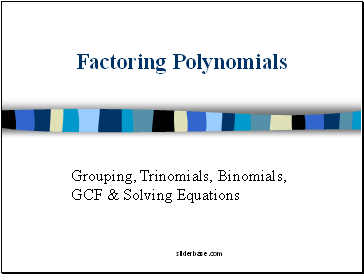Factoring Polynomials

Grouping, Trinomials, Binomials, GCF & Solving Equations

Slide 2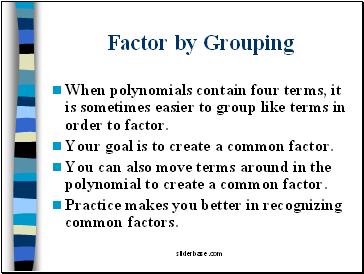## Factor by Grouping

When polynomials contain four terms, it is sometimes easier to group like terms in order to factor.

Your goal is to create a common factor.

You can also move terms around in the polynomial to create a common factor.

Practice makes you better in recognizing common factors.

Slide 3Factoring Four Term Polynomials

Slide 4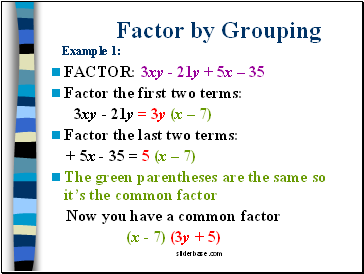Factor by Grouping Example 1:

FACTOR: 3xy - 21y + 5x – 35

Factor the first two terms:

3xy - 21y = 3y (x – 7)

Factor the last two terms:

+ 5x - 35 = 5 (x – 7)

The green parentheses are the same so it’s the common factor

Now you have a common factor

(x - 7) (3y + 5)

Slide 5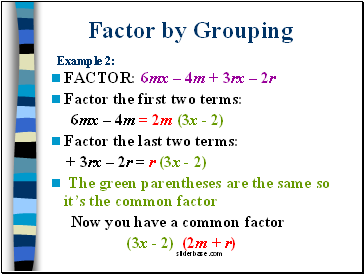Factor by Grouping Example 2:

FACTOR: 6mx – 4m + 3rx – 2r

Factor the first two terms:

6mx – 4m = 2m (3x - 2)

Factor the last two terms:

+ 3rx – 2r = r (3x - 2)

The green parentheses are the same so it’s the common factor

Now you have a common factor

(3x - 2) (2m + r)

Slide 6Factor by Grouping Example 3:

FACTOR: 15x – 3xy + 4y –20

Factor the first two terms:

15x – 3xy = 3x (5 – y)

Factor the last two terms:

+ 4y –20 = 4 (y – 5)

The green parentheses are opposites so change the sign on the 4

- 4 (-y + 5) or – 4 (5 - y)

Now you have a common factor

(5 – y) (3x – 4)

Slide 7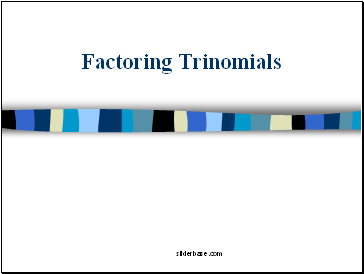## Factoring Trinomials

Slide 8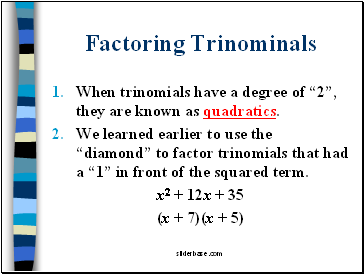Factoring Trinominals

When trinomials have a degree of “2”, they are known as quadratics.

We learned earlier to use the “diamond” to factor trinomials that had a “1” in front of the squared term.

x2 + 12x + 35

(x + 7)(x + 5)

Slide 9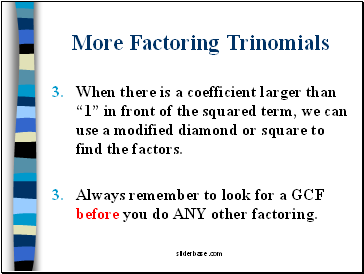More Factoring Trinomials

When there is a coefficient larger than “1” in front of the squared term, we can use a modified diamond or square to find the factors.

Always remember to look for a GCF before you do ANY other factoring.

Slide 10More Factoring Trinomials

Let’s try this example

3x2 + 13x + 4

Go to page:
1  2  3